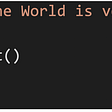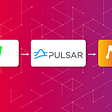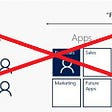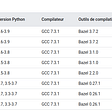# A cheat sheet for custom TensorFlow layers and models

`TensorFlow v2.5.0.`

# Numpy mappings to TensorFlow

• Keep in mind for all operations: in TensorFlow, the first axis is the batch_size.
• Trick to get past the batch size when it’s inconvenient: wrap linear algebra in `tf.map_fn` , e.g. converting vectors of size `n` in a batch `(batch_size x n)` to diagonal matrices of size `(batch_size x n x n)` :
`mats = tf.map_fn(lambda vec: tf.linalg.tensor_diag(vec), vecs)`
• Sum an array: `np.sum(x)``tf.map.reduce_sum(x)`
• Dot product of two vectors: `np.dot(x,y)``tf.tensordot(x,y,1)`
• Matrix product with vector `A.b` : `np.dot(A,b)``tf.linalg.matvec(A,b)`
• Trigonometry: `np.sin``tf.math.sin`
• Transpose `(batch_size x n x m)``(batch_size x m x n)` : `np.transpose(x, axes=(0,2,1))``tf.transpose(x, perm=[0,2,1])`
• Vector to diagonal matrix: `np.diag(x)``tf.linalg.tensor_diag(x)`
• Concatenate matrices: `np.concatenate((A,B),axes=0)``tf.concat([A,B],1)`
• Matrix flatten: `[[A,B],[C,D]]` into a single matrix:
`tmp1 = tf.concat([A,B],2)tmp2 = tf.concat([C,D],2)mat = tf.concat([tmp1,tmp2],1)`
• Kronecker product of a vector `vec` of size `n` (makes a matrix `n x n`): `tf.tensordot(vec,vec,axes=0)` except the vectors usually come in a batch `vecs` of size `(batch_size x n)` , so we need to map:
`mats = tf.map_fn(lambda vec: tf.tensordot(vec,vec,axes=0),vecs)`
• Zeros: `np.zeros(n)``tf.zeros_like(n)` . Note that to avoid the error “Cannot convert a partially known TensorShape to a Tensor”, you should use `tf.zeros_like` instead of `tf.zeros` since the former does not require the size to be known until runtime, see also here.

# Part assignment by indexes

`a = np.random.rand(3)a = 5.0`
`a = tf.Variable(initial_value=np.random.rand(3),dtype='float32')a = 5.0# TypeError: 'ResourceVariable' object does not support item assignment`
`e = tf.one_hot(indices=0,depth=3,dtype='float32')# <tf.Tensor: shape=(3,), dtype=float32, numpy=array([1., 0., 0.], dtype=float32)>`
`a = a + (new_val - old_val) * e`

# What do I need to implement to subclass a Layer?

• Obviously call `super` in the constructor.
• Obviously implement the `call` method.
• You should implement `get_config` and `from_config` — they are needed often for saving the layer, e.g. if `save_traces=False` in the model.
• `@tf.keras.utils.register_keras_serializable(package="my_package")` should be added at the top of the class. From the docs:
`loaded_1 = keras.models.load_model(   "my_model", custom_objects={"MyLayer": MyLayer})`

# What do I need to implement to subclass a Model?

`model.save("model", save_traces=False)# Or just save the weightsmodel.save_weights("model_weights")`
`model = tf.keras.models.load_model("model")# Or just load the weights for an already existing modelmodel.load_weights("model_weights")`
`@tf.keras.utils.register_keras_serializable(package="my_package")`

# How to reduce the size of TensorBoard callbacks if you have a large custom model

`logdir = os.path.join("logs",datetime.datetime.now().strftime("%Y%m%d-%H%M%S"))tensorboard_callback = tf.keras.callbacks.TensorBoard(logdir,   histogram_freq=1,   write_graph=False   )`
`val_checkpoint = tf.keras.callbacks.ModelCheckpoint(   'trained',   monitor='val_loss',   verbose=1,   save_best_only=True,   save_weights_only=True,   mode='auto',   save_frequency=1   )`

# Conclusion

--

--

--

## More from Oliver K. Ernst, Ph.D.

Coding, ML, AI — oliver-ernst.com

Love podcasts or audiobooks? Learn on the go with our new app.

## How to split any string into a list using Python?## Testing Strategy in Android — Part 1## Connect to a Pulsar Cluster and Analyze Streaming NFT Data with Memgraph## Dynamics 365-integrate without requiring a licensed user## Add data to your database with a REST API in just few lines of code## C# preprocessor directives for conditional compiling## Short glimpse of the new Dabble Lab Developer Console !## Installing Anaconda for multiple users## Oliver K. Ernst, Ph.D.

Coding, ML, AI — oliver-ernst.com

## From scratch to CUDA installation and TensorFlow compilation from the sources on Ubuntu 20.04## Fundamentals of Particle Swarm Optimization## Newton-type Methods for Optimization